On this page, you will find Work, Power And Energy Class 9 Notes Science Chapter 11 Pdf free download. CBSE NCERT Class 9 Science Notes Chapter 11 Work, Power And Energy will seemingly help them to revise the important concepts in less time.

## CBSE Class 9 Science Chapter 11 Notes Work, Power And Energy

### Work, Power And Energy Class 9 Notes Understanding the Lesson

1. Work done by a constant force
Work done by a force acting on an object is equal to the magnitude of the force multiplied by the distance moved in the direction of the force.
Work done = force x displacement• Work done has only magnitude and no direction i.e., work is a scalar quantity.
• SI unit of work is joule (J).
• 1 joule (one joule)

W = Fs
If F = 1 N and s = 1 m
W= 1 N x 1 Nm
W =  1 Nm
1j =1 Nm
1 J is the amount of work done on an object when a force of 1 N displaces it by 1 m along the line of action of the force.

2. Conditions that need to be satisfied for work to be done

• Force should act on an object.
• The object must be displaced.

3. Zero work, Positive and Negative work
(i) Zero work: If the angle between force and displacement is 90°, then work done is said to be zero work.
Example: When a man carries a load on his hand and moves on a level road, work done by the man on the load is zero.(ii) Positive work: Work done is said to be positive if force applied on an object and displacement are in the same direction.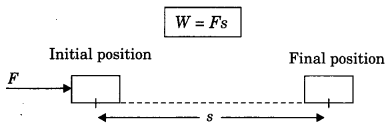Example: Work done by the force of gravity on a falling body is positive.

(iii) Negative work: Work done is said to be negative if the applied force on an object and displacement are in opposite direction.
W = -Fs
Here displacement is taken to be negative (-s).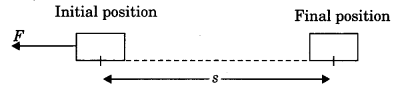Example: Work done by friction force is usually negative on a moving body.

4. Energy
Energy of a body is defined as the capacity or ability of a body to do work.
The SI unit of energy is joule (J) (unit of energy and work is same).

5. Forms of energy
There are various forms of energy in the nature, few of them are mechanical energy (potential energy + kinetic energy) heat energy, chemical energy and light energy.

6. Mechanical energy
Mechanical energy includes kinetic energy and potential energy.

7. Kinetic energy
The energy possessed by a body by the virtue of its motion is called kinetic energy.
Kinetic energy possessed by a body can be calculated by
$$E_{K}=\frac{1}{2} m v^{2}$$
m = mass of body
V = velocity of body

8. Derivation of kinetic energy (work energy theorem)
Let us consider an object lying on a frictionless surface having mass ‘m’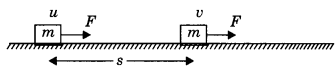A force of constant magnitude F is acting on the body. Here initial velocity of the body is u and final velocity is v. As there is no dissipative forces, work done on the body will be stored in the form of change in kinetic energy.
W=Fs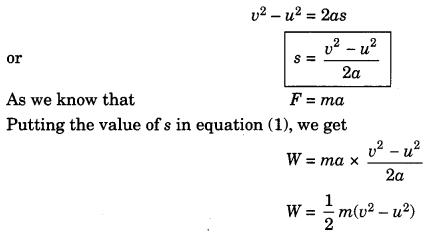If the object is starting from a stationary position u = 0, then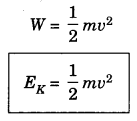9. Potential energy
The energy possessed by a body due to its position or configuration is called potential energy.

10. Gravitational potential energy
Potential energy at any height (h) from a reference can be calculated by formula
Ep = mgh
where, m = mass of object
v = height from reference
The gravitational potential energy of an object at a point above the ground is defined as the work done in raising it from the ground to that point against gravity.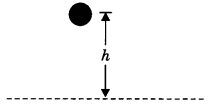11. Derivation of potential energy
When work is done on the body, the work is stored in the form of energy. Consider an object of mass, m. Let it be raised through a height, h from the ground. A force is required to do this. The minimum force required to raise the object is equal to the weight of the object, mg. The object gains energy equal to the work done on it. Let the work done on the object against gravity be W.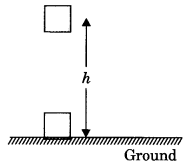That is W = force x displacement
= mgh .
Since work done on the object is equal to mgh, an energy equal to mgh units is gained by the object. This is the potential energy (Ep) of the object.
Ep = mgh

12. Law of conservation of energy
Energy can neither be created nor be destroyed, it can only be transformed from one form to another. The total energy before and after the transformation always remains constant.

13. Transformation of energy in nature
The change of one form of energy into another form of energy is known as transformation of energy.
Example:

• Potential energy of water is converted into electricity in dams.
• Electricity is converted into heat energy in heaters.
• Chemical energy of fuel is converted into mechanical energy in engines.

14. Conservation of mechanical energy
Mechanical energy is the sum of kinetic energy and potential energy.
If there is no loss, then mechanical energy of a system is always constant.
Potential energy + kinetic energy = constant.
or
$$m g h+\frac{1}{2} m v^{2}=\text { constant }$$

15. Power (P)
Power is defined as the rate of doing work or rate of transfer of energy.
Power = work/time
P=W/T

• Unit of power is watt (W).

16. Watt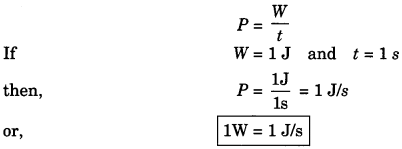17. Commercial unit of energy
Kilowatt hour (kWh) or 1 unit
The energy used in households, industries and commercial establishments are usually expressed in kilowatt hour.
1 kWh is the energy used in one hour (1 h) at the rate of 1000 J/s or (1 kW).
∴1 kWh =lkWxU = 1000 W x 3600 s = 3600000 J
1 kWh = 3.6 x 106 J = 1 unit

18. Power can also be represented as,
P = Fv
F = force applied
v = velocity of object
$$P=\frac{W}{t}=\frac{F s}{t}=F v$$

### Class 9 Science Chapter 11 Notes Important Terms

Work done: Work done by a force acting on an object is equal to the magnitude of the force multiplied by the distance moved in the direction of the force.

Energy: Energy of a body is defined as the capacity or ability of the body to do work.

Mechanical energy: Mechanical energy of a body is the sum of its kinetic energy and potential energy. Kinetic energy: The energy possessed by a body by the virtue of its motion.

Potential energy: The energy possessed by a body due to its position or configuration.

Law of conservation of energy: Energy can neither be created nor be destroyed, it can only be transformed from one form to another.

Conservation of mechanical energy: If there is no loss of energy, then mechanical energy of a system is always constant.

Power: Power is defined as the rate of doing work or rate of transfer of energy.

Commercial unit of energy: The energy used in households, industries and commercial establishment are usually expressed, in kilowatt hour. 1 kWh = 1 unit = 3.6 x 106